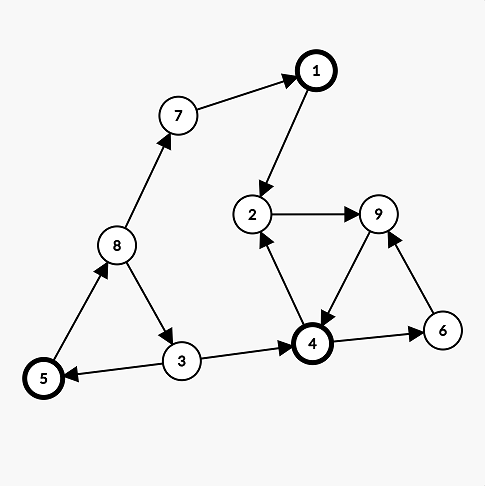시간 제한메모리 제한제출정답맞힌 사람정답 비율
1 초 512 MB125413537.234%

## 문제

Each of Farmer John's $N$ cows ($1\le N\le 2\cdot 10^5$) has a favorite color. The cows are conveniently labeled $1\ldots N$ (as always), and each color can be represented by an integer in the range $1\ldots N$.

There exist $M$ pairs of cows $(a,b)$ such that cow $b$ admires cow $a$ ($1\le M\le 2\cdot 10^5$). It is possible that $a=b$, in which case a cow admires herself. For any color $c$, if cows $x$ and $y$ both admire a cow with favorite color $c$, then $x$ and $y$ share the same favorite color.

Given this information, determine an assignment of cows to favorite colors such that the number of distinct favorite colors among all cows is maximized. As there are multiple assignments that satisfy this property, output the lexicographically smallest one (meaning that you should take the assignment that minimizes the colors assigned to cows $1\ldots N$ in that order).

## 입력

The first line contains $N$ and $M$.

The next $M$ lines each contain two space-separated integers $a$ and $b$ ($1\le a,b\le N$), denoting that cow $b$ admires cow $a$. The same pair may appear more than once in the input.

## 출력

For each $i$ in $1\ldots N$, output the color of cow $i$ in the desired assignment on a new line.

## 예제 입력 1

9 12
1 2
4 2
5 8
4 6
6 9
2 9
8 7
8 3
7 1
9 4
3 5
3 4


## 예제 출력 1

1
2
3
1
1
2
3
2
3


## 힌트

In the image below, the circles with bolded borders represent the cows with favorite color 1.